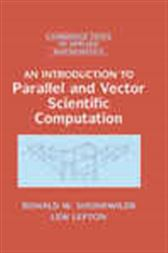An Introduction to Parallel and Vector Scientific Computation

Series: Cambridge Texts in Applied Mathematics (No. 41)US\$45.00
In this text, students of applied mathematics, science and engineering are introduced to fundamental ways of thinking about the broad context of parallelism. The authors begin by giving the reader a deeper understanding of the issues through a general examination of timing, data dependencies, and communication. These ideas are implemented with respect to shared memory, parallel and vector processing, and distributed memory cluster computing. Threads, OpenMP, and MPI are covered, along with code examples in Fortran, C, and Java. The principles of parallel computation are applied throughout as the authors cover traditional topics in a first course in scientific computing. Building on the fundamentals of floating point representation and numerical error, a thorough treatment of numerical linear algebra and eigenvector/eigenvalue problems is provided. By studying how these algorithms parallelize, the reader is able to explore parallelism inherent in other computations, such as Monte Carlo methods.

In The Press

'… in spite of the clear need to present these concepts to a much broader technical audience, there is a perplexing dearth of training material and textbooks in the field, particularly at the introductory level. … it is indeed refreshing to see the publication of the book An Introduction to Parallel and Vector Scientific Computing, written by Ronald W. Shonkwiler and Lew Lefton, both of the Georgia institute of Technology. They have taken the bull by the horns and produced a book that appears to be entirely satisfactory as an introductory textbook for use in such a course.' Scientific Programming